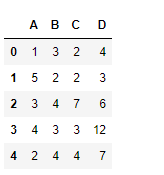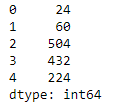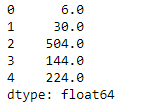Open in App
Not now

# Python | Pandas dataframe.product()

• Last Updated : 22 Nov, 2018

Python is a great language for doing data analysis, primarily because of the fantastic ecosystem of data-centric python packages. Pandas is one of those packages and makes importing and analyzing data much easier.

Pandas` dataframe.product()` function return the value of the product for the requested axis. It multiplies all the element together on the requested axis. By default the index axis is selected.

Syntax: DataFrame.product(axis=None, skipna=None, level=None, numeric_only=None, min_count=0, **kwargs)

Parameters :
axis : {index (0), columns (1)}
skipna : Exclude NA/null values when computing the result.
level : If the axis is a MultiIndex (hierarchical), count along a particular level, collapsing into a Series
numeric_only : Include only float, int, boolean columns. If None, will attempt to use everything, then use only numeric data. Not implemented for Series.
min_count : The required number of valid values to perform the operation. If fewer than min_count non-NA values are present the result will be NA.

Returns : prod : Series or DataFrame (if level specified)

Example #1: Use `product()` function to find product of all the elements over the column axis in the dataframe.

 `# importing pandas as pd ` `import` `pandas as pd ` ` `  `# Creating the dataframe  ` `df ``=` `pd.DataFrame({``"A"``:[``1``, ``5``, ``3``, ``4``, ``2``],  ` `                   ``"B"``:[``3``, ``2``, ``4``, ``3``, ``4``],  ` `                   ``"C"``:[``2``, ``2``, ``7``, ``3``, ``4``],  ` `                   ``"D"``:[``4``, ``3``, ``6``, ``12``, ``7``]}) ` ` `  `# Print the dataframe ` `df `Let’s use the `dataframe.product()` function to find the product of each element in the dataframe over the column axis.

 `# find the product over the column axis ` `df.product(axis ``=` `1``) `

Output :Example #2: Use `product()` function to find the product of any axis in the dataframe. The dataframe contains `NaN` values.

 `# importing pandas as pd ` `import` `pandas as pd ` ` `  `# Creating the first dataframe  ` `df ``=` `pd.DataFrame({``"A"``:[``1``, ``5``, ``3``, ``4``, ``2``], ` `                   ``"B"``:[``3``, ``None``, ``4``, ``3``, ``4``],  ` `                   ``"C"``:[``2``, ``2``, ``7``, ``None``, ``4``], ` `                   ``"D"``:[``None``, ``3``, ``6``, ``12``, ``7``]}) ` ` `  `# using prod() function to raise each element ` `# in df1 to the power of corresponding element in df2 ` `df.product(axis ``=` `1``, skipna ``=` `True``) `

Output :My Personal Notes arrow_drop_up
Related Articles